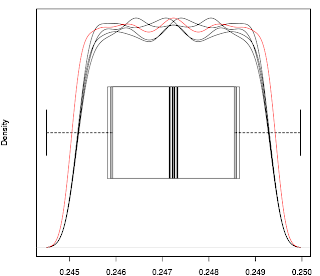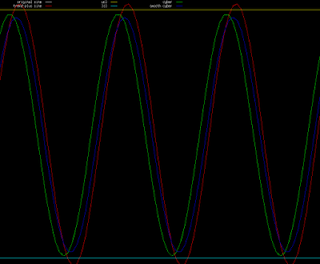## Tuesday, 27 April 2010

### Monte Carlo Optimisation of Control Limits #2Having run the routine again on sine waves of various amplitudes I have plotted boxplots and kernal density plots of the ucl and lcl optimised multipliers for sine waves of periods n=10 to 50 inclusive, using R. Visual inspection of the plots suggest that, in general* (see note below), there are no real differences between the distributions and a typical plot is shown above (again this is for a sine wave of period n=20). The black plots are
• optimised multiplier for ucl of sine wave with amplitude of 1
• optimised multiplier for lcl of sine wave with amplitude of 1
• optimised multiplier for ucl of sine wave with variable amplitude
• optimised multiplier for lcl of sine wave with variable amplitude
The red line is a kernal density plot of all four of the above combined into a single, global distribution for that period sine wave.

Obviously just looking at plots is not statistically robust, so the next thing I did was to run Kolmogorov-Smirnov tests in R to see if the null hypothesis that the four separate distributions come from the same distribution can be rejected. For this purpose I used a p-value of 0.05 for the significance level. The comparative test runs were
1. ucl sine wave amplitude 1 and lcl sine wave amplitude 1
2. ucl sine wave variable amplitude and lcl sine wave variable amplitude
3. ucl sine wave amplitude 1 and ucl sine wave variable amplitude
4. lcl sine wave amplitude 1 and lcl sine wave variable amplitude
The logic behind this choice is:-
if A=B and C=D and A=C and B=D then A=B=C=D.

The actual test in R that was used was the Bootstrap Kolmogorov-Smirnov package ks.boot(), the R documentation description for which is quoted below:-

"This function executes a bootstrap version of the univariate Kolmogorov-Smirnov test which provides correct coverage even when the distributions being compared are not entirely continuous. Ties are allowed with this test unlike the traditional Kolmogorov-Smirnov test"

It is obvious from the discrete nature of the raw data being tested that this test is more appropriate than the traditional Kolmogorov-Smirnov test, but more importantly the symmetrical nature of a sine wave almost guarantees that there are likely to be ties in the data, hence the choice of this test. The number of bootstraps performed for each test was n=1000. The p-value was sufficiently low to reject the null hypothesis for the following periods (numbered bullet points correspond to tests identified above)

1. Periods 16 ,24, 32, 40 and 48
2. Periods 16, 24, 27, 32, 40, 45, 48, 49
3. Periods 38 and 49
4. Periods 38 and 49

*The exceptions to the general pattern mentioned above will be the subject of a later blog entry.

## Sunday, 25 April 2010

### Monte Carlo Optimisation of Control Limits #1The overnight run went without a hitch and I now have a text file of 3000 rows (the number of iterations in the MC routine) and columns for the ucl and lcl multipliers for sine waves of periods 10 to 50 inclusive.

As a first simple analysis of these I have used the simple R commands boxplot(ucl) and plot(density(ucl)) to plot graphs for quick visual examination of the distribution of these multipliers, each graph being either ucl or lcl for one sine wave period only. A generic example of boxplot(ucl) is the first graph above (this happens to be ucl for sine wave period 20). As can be seen, there are no outliers beyond the whiskers and it would appear that the distribution is evenly spread about the thick median line.

The second graph is plot(density(ucl)), a plot of the kernal density. Note the steep drop offs at about 2.00 and 2.15. This is a pattern that repeats for almost all periods for both the ucl and lcl, although of course with different values at the drop off points. This second graph suggests that in fact two multipliers could be used to create a "zone" ucl and lcl. It can be conjectured that
• if prices are outside this zone, either to the upside of the ucl zone or downside of the lcl zone, there would be a high probability that in fact prices are trending,
• whilst if prices are between the zones they could be considered to be in a sideways channel
• whilst if prices are actually between the lines that create either zone they could be considered to be in a high probability area for a price reversal in a sideways market, or alternatively in a zone where a breakout from a sideways market is imminent.
However, before this particular idea is investigated further there is one more run of the MC routine I would like to do. The above results come from a run where the sine wave amplitude is one. Of course real prices, if they are in a sideways channel, won't be oscillating with this amplitude but will have variable amplitudes. Therefore the MC routine shall be run again with sine waves of various amplitudes, and the results compared with those obtained so far.

## Saturday, 24 April 2010

### First step in optimising Tukey Chart

I have now written a simple Monte Carlo routine in Octave script to loop through a series of sine waves of periods 10 to 50 inclusive, with an amplitude of 1, to extract a series of optimised ucl and lcl multipliers such that each multiplier ensures that the ucl and lcl for that particular sine wave has a value of 1, i.e. perfectly encloses said sine wave between the control limits. The code is certainly "clunky" and not optimised for speed, but this is not important as it will only need to be run a few times. I calculate that it will take about 10 hours to obtain a total sample of 3000 multipliers for each sine wave period. I shall run this overnight tonight while I am sleeping and have the results ready for analysis from tomorrow.

## Wednesday, 21 April 2010

### Applying Control Charts to price seriesFor my first substantive post I thought I would post a screenshot (http://www.gnuplot.info) of what I'm currently working on. The red line is a sine wave and the lines of interest are the yellow upper control line (ucl) and the blue lower control line (lcl). For a description of what the ucl and lcl are see http://gunston.gmu.edu/708/frTukey.asp

The basic idea is to apply the concept of a Tukey Control Chart to prices to determine whether prices are moving sideways or not. To that end, the above sine wave models a sideways moving market and represents the pre-intervention period for constructing the ucl and lcl. The idea is that if prices are outside either of the control limits then prices are NOT moving sideways in a cyclic fashion and by default must be either trending up or down. This in turn will determine what trading approach to take given current market conditions and what indicators to use.

The above ucl and lcl do not use Tukey's recommended 1.5 multiplier for the Fourth Spread but instead use a different multiplier depending on the period of the sine wave. This has been determined simply by looking at numerous charts and adjusting the multiplier so that the control lines are as close as possible, visually, to the peaks and troughs of the sine wave. Future work to be done is to write a program/routine - I use Octave (http://www.gnu.org/software/octave) and C++ functions written for Octave, and occasionally the statistical software R (http://www.r-project.org) in my development work - to optimise this multiplier for each period length. For help in coding this I have posted on the Octave Nabble forum and I am waiting for any responses. The hope/expectation is that on a real price chart the ucl and lcl will indicate support and resistance areas when prices are in a sideways moving channel as well as indicating the fact that current price is actually moving in a fashion that, statistically speaking, is indistinguishable from a sideways moving channel.

## Saturday, 17 April 2010

### Reasons for starting this blog

The purpose of this blog is for me to document the development of a trading system that I intend to use for my own trading and to hopefully receive constructive criticism from those who care to offer it. I have been testing different elements of this system on and off and in isolation from each other for some time now on my computer and in "demo account" mode with Collective2 (press clickable Collective2 icon at the top of the blog), with an occasional live trade, and I now want to completely overhaul and improve each element and integrate them so that together they constitute a complete trading system. At the moment I don't envisage that this system will ever be 100% mechanical or fully automated in the sense of a trading robot or EA, but I do hope that this process of development and possible feedback from readers will lead to a set of indicators/rules/guidelines that will prove to be profitable in the future.

Another reason for starting the blog is the idea that it will become my online trading journal.